• ## 状态方程离散化

千次阅读 2019-06-10 14:18:25
由于使用卡尔曼滤波需要离散化的系统状态方程，即系统方程和量测方程都需要是离散型的，而在实际的物理系统中我们得到的是连续系统的状态方程，动力学特性用连续微分方程描述，所以对它们需要进行离散化处理。...
由于使用卡尔曼滤波需要离散化的系统状态方程，即系统方程和量测方程都需要是离散型的，而在实际的物理系统中我们得到的是连续系统的状态方程，动力学特性用连续微分方程描述，所以对它们需要进行离散化处理。在这里我就不放离散化的具体推导，毕竟很多人也只是用这个公式，而不必去真正了解过程，能用就可以了。如果有大佬想了解具体推导过程，可以去看看秦永元等主编的《卡尔曼滤波与组合导航原理》，里面介绍的还是很详细的。

描述物理系统动力学特性的系统方程为：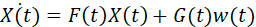其中系统的驱动源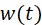为白噪声过程，即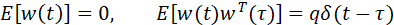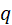为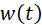的方差强度阵。

根据线性系统理论，系统方程的离散化形式为：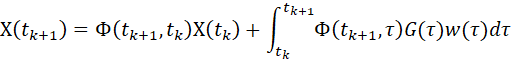其中一步转移阵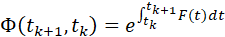当滤波周期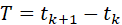较短时，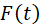可近似看作常阵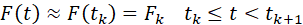此时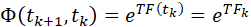即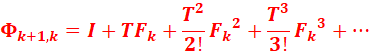一般计算到二阶即可。

令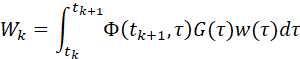则离散后的方程形式为：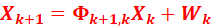其中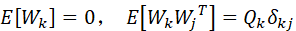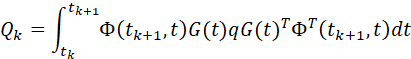当滤波周期k较短时，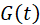在该周期内变化不大，则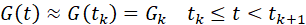令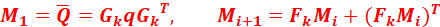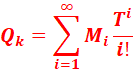计算一步转移阵和等效离散系统噪声方差阵如下图所示：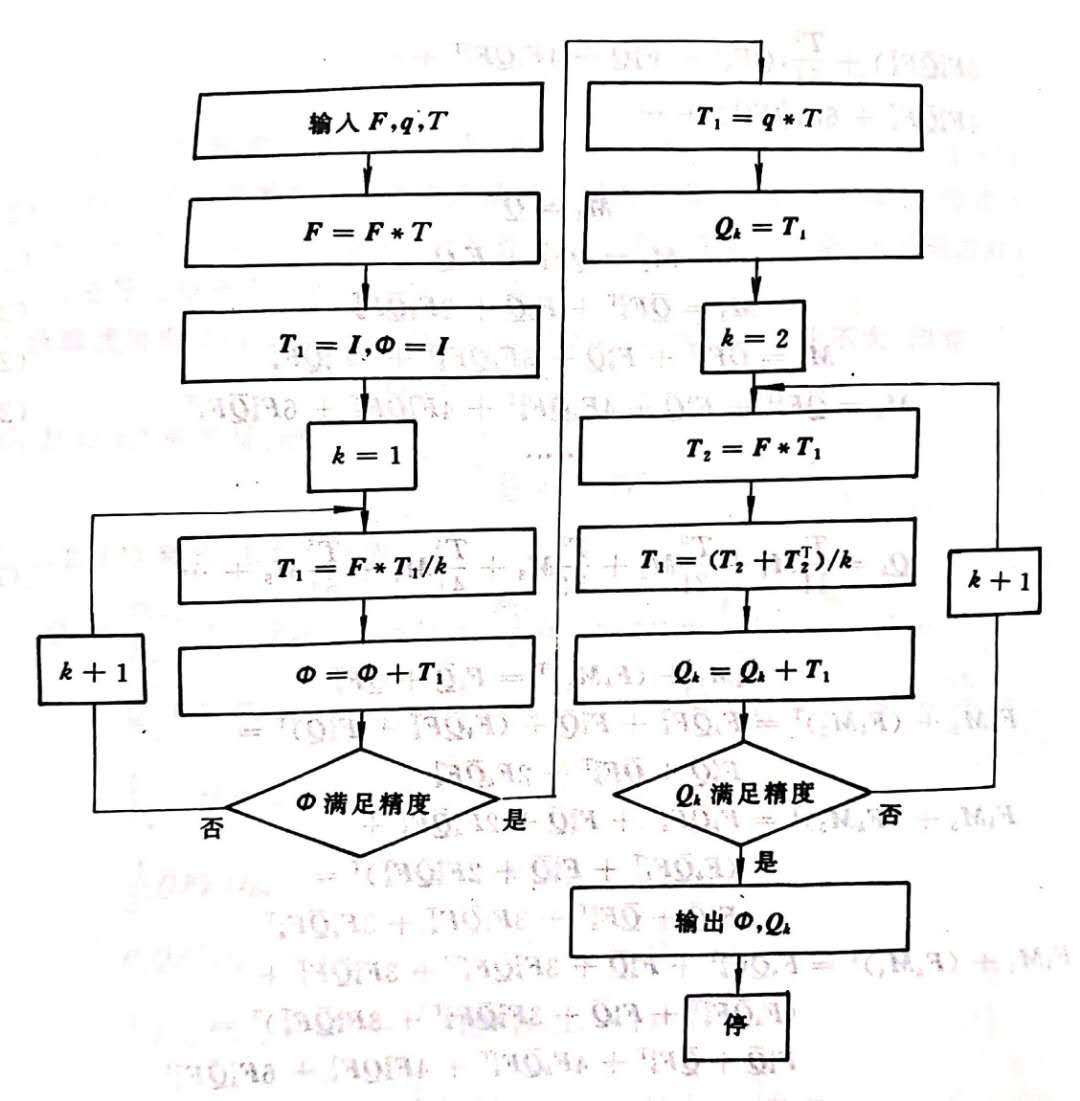若滤波周期较长，采样频率较高，且系统矩阵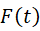随时间变化较剧烈，而在一个滤波周期内能得到N个采样值，则计算一步转移阵和等效离散系统噪声方差阵时可利用这些采样值来简化算法。

设滤波周期为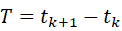，在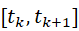内每隔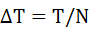就能得到系统阵的采样值：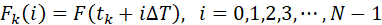一步转移阵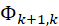和等效离散系统噪声方差阵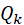可按如下方法简化计算：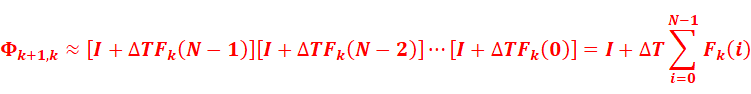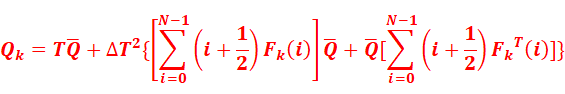考察上式可看出，计算一步转移阵所需矩阵运算仅需作N步矩阵求和，计算等效离散系统噪声方差阵所需的矩阵运算为作N步矩阵求和后作一步矩阵相乘，与之前的公式相比计算量大为减小，对工程实现十分有利。此外，与未简化的算法仅用到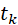时刻系统的动态信息相比，简化算法利用了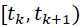内N次采样获得的系统动态信息，这在一定程度上弥补了级数过早截断引起的精度损失。

也可以利用MATLAB直接进行状态方程离散化

在matlab中，[G,H]=c2d(A,B,Ts)，Ts是采样周期

得到离散化状态空间等式：x(k+1)=Gx(k) + Hu(k)

展开全文卡尔曼滤波
• 连续系统转化为离散系统的方法之-- z变换。z变换主要研究如何将连续系统传递函数G(s) 转化为离散传递函数 G(z)。就是找到一个s和z的关系，直接将G(s)中的s全部替换为z便大功告成。 线性连续系统的表达方法之一便是...
差分变换
连续系统转化为离散系统的方法之-- z变换。z变换主要研究如何将连续系统传递函数G(s) 转化为离散传递函数 G(z)。就是找到一个s和z的关系，直接将G(s)中的s全部替换为z便大功告成。
线性连续系统的表达方法之一便是传递函数
$C(s) = \frac{b_0s^{n-1}+...+b_{n-1}}{s^n+a_0s^{n-1}+...+a_{n-1}} \tag{1}$
线性离散系统的表达方法之一也是传递函数，用 Z表示差分
$G(s) = \frac{b_0z^{n-1}+...+b_{n-1}}{z^n+a_0z^{n-1}+...+a_{n-1}} \tag{2}$
注意$zy(z) = y(k+1),z^{-1}y(z) = y(k-1)$
重点，连续系统传递函数(1)中的基本单元是s，离散系统传递函数(2)中基本单元是 z。离散化便是找到一个s和z的对应关系，然后将s替换成z即可。
常用三种对应关系：
1.前项差分变换   (T为采样间隔)
$z = 1+Ts \Rightarrow s = \frac{z-1}{T}$
2.后项差分变换 (T为采样间隔)
$z = \frac{1}{1-Ts} \Rightarrow s = \frac{z-1}{Tz}$
3.Tustin 变换 (双线性变换，常用方法) (T为采样间隔)
$z = \frac{1+\frac{Ts}{2}}{1-\frac{Ts}{2}} \Rightarrow s = \frac{2}{T}\frac{z-1}{z+1}$
离散化案例一：
$G(s)=\frac{s}{s+1}$
$C_{FE}=\frac{\frac{1}{T}(z-1)}{\frac{1}{T}(z-1)+1}=\frac{z-1}{z-1+T}=\frac{1-z^{-1}}{1+(T-1)z^(-1)}$
离散化案例二：二阶巴特沃斯滤波器
如何记住者三种变换：
首先要记住$z=e^{Ts}$(由定义)，再根据泰勒展开，由于采样时间较小，Ts近似为0，略去高次项，可得：
$z=e^{Ts}\approx 1+Ts$
此为前向差分变换
再进一步变换
$z=e^{Ts}=(e^{-Ts})^{-1}\approx (1-Ts)^{-1}$
此为后向差分变换
再进一步变化
$z=e^{Ts}=\frac{e^{\frac{Ts}{2}}}{e^{-\frac{Ts}{2}}}\approx\frac{1+\frac{Ts}{2}}{1-\frac{Ts}{2}}$
欧拉法
我们还会遇到
$\dot{x} = Ax+bu$
对于这种如何进行离散化呢？答案是使用欧拉法。
对上式两边进行积分得：
$\int_{t}^{t+dt}\dot{x}dt=\int_{t}^{t+dt}(Ax+Bu)dt$
上式左边：
$\int_{t}^{t+dt}\dot{x}dt=x(t+dt)-x(t)$
右边根据积分中值定理知道：
$\int_{t}^{t+dt}Axdt=Ax(\xi)dt,其中t< \xi< t+dt$
那么：
向前欧拉法：$x(\xi)=x(t)\Rightarrow x(t+dt)=(I+Adt)x(t)$
向后欧拉法：$x(\xi)=x(t+dt)\Rightarrow x(t+dt)=(I-Adt)^{-1}x(t)$
中点欧拉法：$x(\xi)=\frac{x(t+dt)+x(t)}{2} \Rightarrow x(t+dt)=(I-\frac{Adt}{2})^{-1}(I+\frac{Adt}{2})x(t)$
声明：文章上半部分参考这里，侵删。
参考文章：
https://blog.csdn.net/weixin_39830200/article/details/111366595
https://blog.csdn.net/u013492900/article/details/114884926


展开全文• 假设一个连续状态空间等式为：(x)=Ax+Bu 忽略输出方程，因为离散后的输出方程与连续时一样 在matlab中，[G,H]=c2d(A,B,Ts)，Ts是采样周期 得到离散化状态空间等式：x(k+1)=Gx(k) + Hu(k) ...
假设一个连续状态空间等式为：(x)=Ax+Bu
忽略输出方程，因为离散后的输出方程与连续时一样
在matlab中，[G,H]=c2d(A,B,Ts)，Ts是采样周期
得到离散化状态空间等式：x(k+1)=Gx(k) + Hu(k)


展开全文• 假设连续系统的线性状态方程为： x˙=Ax+bu\dot{ \mathbf{x}}=\mathbf{A}\mathbf{x}+\mathbf{b}ux˙=Ax+bu, x(0)=x0x(0)=x_0x(0)=x0​ 其状态转移矩阵为： Φ(t)=∑k=0∞Aktkk!=eAt\mathbf{\Phi}(t)=\sum_{k=0}^{\...
假设连续系统的线性状态方程为：
$\dot{ \mathbf{x}}=\mathbf{A}\mathbf{x}+\mathbf{b}u$,  $x(0)=x_0$
其状态转移矩阵为：
$\mathbf{\Phi}(t)=\sum_{k=0}^{\infty} \mathbf{A}^{k} \frac{t^{k}}{k !}=e^{\mathbf{A} t}$
其非齐次微分方程的解为：
$\mathbf{x}(t)=\underbrace{\mathbf{\Phi}\left(t-t_{0}\right) \mathbf{x}\left(t_{0}\right)}_{\text {齐次解 }}+\underbrace{\int_{t_{0}}^{t} \mathbf{\Phi}(t-\tau) \mathbf{b} u(\tau) d \tau}_{\text {特解 }}$
令$t_0:=t_k=kT$，并且$t:=t_{k+1}=(k+1)T$，则有
$\mathbf{x}\left(t_{k+1}\right)=\mathbf{\Phi}(T) \mathbf{x}\left(t_{k}\right)+\int_{t_{k}}^{t_{k+1}} \Phi\left(t_{k+1}-\tau\right) \mathbf{b} u(\tau) d \tau$
因为采样保持，在一个采样区间里，输入控制变量恒定$u(\tau)=u_k=\text{const}$，$t \in[t_k,t_{k+1})$，所以上式$u(\tau)$可以提出：
$\mathbf{x}\left(t_{k+1}\right)=\mathbf{\Phi}(T) \mathbf{x}\left(t_{k}\right)+\int_{t_{k}}^{t_{k+1}} \Phi\left(t_{k+1}-\tau\right) \mathbf{b} d \tau u_k$
换元，$t_{k+1}-\tau=\kappa$，所以$d\kappa = d(t_{k+1}-\tau)=-d\tau$
$\mathbf{x}\left(t_{k+1}\right)=\mathbf{\Phi}(T) \mathbf{x}\left(t_{k}\right)+\int_{T}^{0} -\Phi\left( \kappa \right) \mathbf{b} d \kappa u_k$
$\mathbf{x}\left(t_{k+1}\right)=\mathbf{\Phi}(T) \mathbf{x}\left(t_{k}\right)+\int_{0}^{T} \Phi\left( \kappa \right) \mathbf{b} d \kappa u_k$
比较离散状态方程$\mathbf{x}(t_{k+1})=\mathbf{A}_d\mathbf{x}(t_k)+\mathbf{b}_d u_k$
$\mathbf{A}_{d}=\mathbf{\Phi}(T)=\sum_{k=0}^{\infty} \mathbf{A}^{k} \frac{T^{k}}{k !}=e^{\mathbf{A} T}$
$\mathbf{b}_{d}=\int_{0}^{T} \mathbf{\Phi}(\kappa) \mathbf{b} d \kappa$
$\mathbf{c}_{d}^{\mathrm{T}}=\mathbf{c}^{\mathrm{T}}$
离散的动态系数矩阵为指数矩阵，求解方法为连续矩阵求和，只有当$k\to\infty$ 能够取到无穷阶才有完全精确的表达。自然和连续的动态矩阵$\mathbf{A}$不一样。
如果是非线性系统，没有微分方程的通解，那也可以采用同样的思路，对非线性的状态方程采取欧拉法，乃至龙格库塔法逼近。
以上转自知乎用户 善道 在问题如何理解状态空间中状态方程系数矩阵的离散化后的结果？下的回答，仅作个人笔记使用。
当系统带有扰动时，系统的状态方程为：
$\dot{ \mathbf{x}}=\mathbf{A}\mathbf{x}+\mathbf{b}u+\mathbf{d}(t)$,  $x(0)=x_0$
同样采用知乎用户 善道 的方法
$\mathbf{x}\left(t_{k+1}\right)=\mathbf{\Phi}(T) \mathbf{x}\left(t_{k}\right)+\int_{t_{k}}^{t_{k+1}} \Phi\left(t_{k+1}-\tau\right) \mathbf{b} d \tau u_k+\int_{t_{k}}^{t_{k+1}} \Phi\left(t_{k+1}-\tau\right)\mathbf{d}(\tau)d\tau$
换元，$t_{k+1}-\tau=\kappa$
$\mathbf{x}\left(t_{k+1}\right)=\mathbf{\Phi}(T) \mathbf{x}\left(t_{k}\right)+\int_{0}^{T} \Phi\left( \kappa \right) \mathbf{b} d \kappa u_k +\int_{0}^{T} \Phi\left( \kappa \right) \mathbf{d}\left( \left(k+1 \right)T-\kappa \right) d \kappa$


展开全文• 如果用数字计算机对连续时间状态方程求解，或者对连续受控对象采用数字计算机进行在线控制，都要碰到一个将连续时间系统化为离散时间系统的问题。本节将讨论线性连续时间状态空间表达式的离散化方法。
• 首先对模糊规则后件部分的线性时不变动态方程做局部离散化处理，然后再对系统的动态模型做全局离散化处理。为了获得T-S模糊系统的多胞结构，对全局离散化模型进行Taylor级数展开，从而得到近似全局离散化模型。基于...
• N个连续的盒子，每个盒子有高度h和价值v，选择任意一点进入，且从...状态转移方程：dp[i]=max(dp[j])+v[i], (0h[j]) 用线段树优化，寻找 j #include "stdio.h" #include "string.h" #include "queue" #inc
• 在给出综合估计法模型的基础上，对估计方法连续状态方程离散化，并给出闭环状态观测器反馈阵以及离散模型系数矩阵的计算方法。试验结果表明：综合估计法能充分汲取直接积分法的非线性不敏感和模型估计法无累积误差的...
• 线性系统的状态空间分析法 迭代程序法 iterative program method 线性连续状态方程离散化
• 首先，建立了相邻结构体系的力学模型及连续运动状态方程，并将其离散化成标准离散状态方程形式。其次，简要地讨论了离散变结构控制器的设计方法，包括切换平面的选择及离散趋近率的构成。最后，应用本文方法对主楼12...
• 线性控制系统状态空间表达式的求解2.1 线性定常连续系统齐次状态方程的解2.2 线性定常连续系统的状态转移矩阵2.3 线性定常连续系统非齐次状态方程...离散时间系统状态方程式的求解2.6 线性连续时间系统的离散化2.7 利用...
• 差分方程模型系列： 差分方程模型（一）：模型介绍与Z变换 差分方程模型(二）：蛛网...通过解差分方程来求微分方程的近似解，是连续问题离散化的一个例子。 离散状态转移模型涉及的范围很广，可以用到各种不同的...
• 误差状态的运动方程2.1连续时间的IMU系统动态方程2.1.1相关变量2.1.2真实状态运动方程(true-state kinematics equations )2.1.3标称状态运动方程(normal-state kinematics equations )2.1.4误差状态...
• 1. 引言 1.1微分方程是描述一个系统的状态随时间和空间变化的最基本的数学表达式，在物理，经济，工程等方面都有及其重要的...首先将连续变量y和t离散化连续的函数y(t)和f(y,t)化为离散的 yny_{_{n}}yn​​和f(yn,tpython 算法
• 文章目录1....误差状态的运动方程2.1连续时间的IMU系统动态方程2.1.1相关变量2.1.2真实状态运动方程(true-state kinematics equations )2.1.3标称状态运动方程(normal-state kinematics equations )2.1.4...
• 数学上求解数值解 利用数学理论推导的方法求得微分方程解称为解析解或者精确解，其特点是得到一个状态变量关于自变量的函数关系表达式。...首先 把区间离散化： [a,b] 变成离散的点： 同时 记： ...matlab ode
• 根据它们之间的关系，采用离散化数字解算法求解，即将目标函数、状态方程和约束变量方程等都离散化，形成迭代循环，从而得到切换时刻的脉冲优化数字解，保证了系统轨迹在约束范围内达到优化状态。
• 新算法通过连续系统离散化获得融合周期内各采样点同融合时刻之间状态的动态关系,并利用该关系来建立相邻两个采样时刻间符合标准Kal-man滤波条件的状态递归方程以及相应的测量方程,然后通过执行顺序Kalman滤波来实现...
• 采用零阶保持采样，将方程离散化。推导了GPS与INS闭环积分的扩展卡尔曼滤波器。滤波器对误差状态进行传播和估计，并将误差状态反馈给惯性导航系统进行内部导航状态校正。该算法在上位机上实现，上位机通过串口接收...
• 基于五阶段超混沌电路系统、数字化处理技术，通过对系统的连续时间状态方程进行离散化处理和变量比例变换，用FPGA技术硬件实现超混沌电路系统方程中的混沌吸引子；给出了实现混沌吸引子的FPGA技术，指出了硬件实现的...
• 系统的数学模型可以用多种相互等价的数学方程形式来表达，例如微分方程组与连续状态方程、差分方程组与离散状态方程等等，对于线性时不变系统还经常在变换域中来描述，例如频域、复频域和Z域描述等等。对于单输入单...
• 对坐标系及其转换关系进行了定义，推导构建了基于加表、陀螺和GPS的组合姿态测量系统测量元件模型和全量模型，通过状态方程和测量方程的分析推导对全量模型进行了线性化处理，并对连续系统模型作了离散化处理。...
• 前言： 线性系统理论是控制专业的一门专业基础课。所需基础：现代控制理论 和 计算机控制技术。 1.线性系统的数学描述 线性系统的输入输出描述 线性系统的状态空间 ... 线性连续系统的时间离散化...李亚普诺夫
• 首先,对Lorenz连续系统的方程进行分解,得到离散化状态方程,接着基于DSP-Builder软件开发平台获得系统的电路模型,该模型可直接转化为VHDL语言;其次,采用硬件描述语言( Verilog HDL)直接编程的形式,对系统进行验证,并...
• 第4章 连续系统按环节离散化的数字仿真 4.1 连续系统的离散化 4.2 典型环节的离散系数及其差分方程 4.3 非线性系统的数字仿真方法 4.4 连续系统按环节离散化的数字仿真程序 第5章 采样控制系统的数字仿真 5.1 ...
• 2、线性连续系统的离散化 二、理论推导 画重点：当考虑系统受到载荷激励时，微分方程右边为载荷激励；当考虑系统受到位移和速度的激励时，右边为位移速度激励以刚度k和阻尼c为系数的线性合作。这是两个不同的动力学...算法
• 采用零阶保持采样，将方程离散化。推导了GPS与INS闭环积分的扩展卡尔曼滤波器。滤波器对误差状态进行传播和估计，并将误差状态反馈给惯性导航系统进行内部导航状态校正。该算法在上位机上实现，上位机通过串口接收...
• 高精度的动态模型是实现小型无人直升机高性能自主飞行控制的基础。给出了在灰箱建模框架下辨识...辨识步骤在离散时域中进行，利用sinch算法将连续时域的状态空间参数问题映射到离散域。提出的小型无人直升机灰箱建
• （3）s-function是以现代控制理论的状态方程为基础的 二、如何编写一个自己的s-function （1）介绍 支持m函数语言、C语言、C++语言、和Fortran 模板函数是s－function的框架，以下会介绍 S－function的参数有...simulink
• 代替指定离散的隐藏层序列，我们使用神经网络对隐藏状态的导数进行参数。 我们还构造了连续规范流，这是一种生成模型，可以最大程度地进行训练，而无需对数据维进行分区或排序 解剖神经ODE（口头）： 连续...